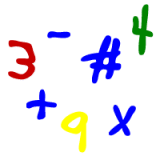Parents and Teachers: Support Ducksters by following us onor.

# Kids Math

## Order of OperationsSkills needed:
Multiplication
Division
Addition
Subtraction

In math problems it's important to do the operations in the right order. If you don't, you may end up with the wrong answer. In math, there can be only one correct answer, so mathematicians came up with rules to follow so we can all come up with the same correct answer. The correct order in math is called the "order of operations". The basic idea is that you do some things, like multiplication, before others, like addition.

For example, if you have 3 x 2 + 7 = ?

This problem could be solved two different ways. If you did the addition first you would get:

3 x 2 + 7
3 x 9 = 27

If you do the multiplication first, you get:

3 x 2 + 7
6 + 7 = 13

The second way is correct as you should do the multiplication first.

Here are the rules in the Order of Operations:
• Do everything inside of brackets first.
• Next, any exponents or roots (if you don't know what these are, don't worry about them for now).
• Multiplication and division, performing them left to right
• Addition and subtraction, performing them left to right
Let's do a few examples:

40 + 1 - 5 x 7 + 6 ÷ (3 x 2)

First we do the brackets:

40 + 1 - 5 x 7 + 6 ÷ 6

Now we do the multiplication and division, left to right:

40 + 1 - 35 + 1

Now addition and subtraction, left to right:

The answer = 7

Note: even on the last step if we had added 35 + 1 first then we would have done 41 - 36 = 5. This is the wrong answer. So we need to do the operations in order and left to right.

Another order of operations example:

6 x 12 - (12 x 7 - 10) + 2 x 30 ÷ 5

We do the math inside the brackets first. We do the multiplication in the brackets first:

6 x 12 - (84 - 10) + 2 x 30 ÷ 5

Finish the brackets:

6 x 12 - 74 + 2 x 30 ÷ 5

Multiplication and division next:

72 - 74 + 60 ÷ 5
72 - 74 + 12

The answer is 10.

How to remember the order?

There are different ways to remember the order. One way is to use the word PEMDAS. This can be remembered by the phrase "Please Excuse My Dear Aunt Sally". What it means in the Order of Operations is "Parentheses, Exponents, Multiplication and Division, and Addition and Subtraction". When using this you must remember that multiplication and division are together, multiplication doesn't come before division. The same rule applies to addition and subtraction.

More Algebra Subjects
Algebra glossary
Exponents
Linear Equations - Introduction
Linear Equations - Slope Forms
Order of Operations
Ratios
Ratios, Fractions, and Percentages
Solving Algebra Equations with Addition and Subtraction
Solving Algebra Equations with Multiplication and Division

Back to Kids Math

Back to Kids StudyAbout Ducksters Privacy Policy

Follow us onorThis site is a product of TSI (Technological Solutions, Inc.), Copyright 2019, All Rights Reserved. By using this site you agree to the Terms of Use.